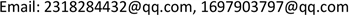1. 引言

Tarski在1948年的一篇经典著作  中，解决了闭实数域的判定问题，其主要目的之一，是给出初等几何的机械化证明。以后Seidenberg  ，Robinson  与Cohen  等人又给出了Tarski定理的不同证法。同时这些作者也给出了初等几何定理机械化证明的一些建议，但这些建议离真正的机械化证明甚远，最终由中国科学家吴文俊院士在1977年文献  中给出了一种初等几何定理机械化证明的一种设计方法——吴方法。吴方法主要是引进笛卡尔直角坐标系，即引进坐标，然后代数化，这里条件和结论都进行代数化，即用条件代数方程组去推出结论代数方程式，从而对命题判断真伪。这里条件代数方程组运用的是吴文俊消元法进行得出结论代数方程式，从而实现定理的机械化证明。2014年初周亚南在文献  中发明了一种新的消元法，从而试想用这种新的消元法对定理的机械化证明一个大的改进，使机械化证明不在单一化，而是多元化，不仅仅世上仅存吴方法。而周亚南在文献  的论述与一些猜想和问题更使我们认为定理的机械化证明可以用新的消元法来实现。当然，这里同样离不开笛卡尔直角坐标系，同样需要对线段的长度，以及垂直关系，平行关系，相等关系等进行代数化，这里由于在文献  中吴文俊院士已经给出，我们这里省略，需要者可以查阅文献  。还有是否能够用吴方法或者本文的消元技术对文献  进行数学的机械化证明，当然如果能够对文献  进行定理的机械化证明这将是科学的一大进步。最后，能否将本文的方法推广到数学的其他科目上面，比如微分几何定理的机械化证明，对于文献  中的消元技术能否应用到代数几何以及曲面造型等上面等，由于篇幅原因，本文作者仅给出一个例子，即勾股定理的机械化证明，希望读者群体敬请谅解。

2. 命题与一些必要的条件和结论2.1. 命题

(1)结论

2.2. 条件

A B 2 = ( x 2 − x 1 ) 2 + ( y 2 − y 1 ) 2 (2)条件

A C 2 = ( x 3 − x 1 ) 2 + ( y 3 − y 1 ) 2 (3)条件

(4)条件

( y 3 − y 2 ) ( y 3 − y 1 ) + ( x 3 − x 2 ) ( x 3 − x 1 ) = 0 (5)条件

A B 2 = x 1 2 + x 2 2 + y 1 2 + y 2 2 − 2 x 1 x 2 − 2 y 1 y 2 (2a)变形条件

A C 2 = x 1 2 + x 3 2 + y 1 2 + y 3 2 − 2 x 1 x 3 − 2 y 1 y 3 (3a)变形条件

B C 2 = x 2 2 + x 3 2 + y 2 2 + y 3 2 − 2 x 2 x 3 − 2 y 2 y 3 (4a)变形条件

y 3 2 + y 1 y 2 + x 3 2 + x 1 x 2 − y 1 y 3 − y 2 y 3 − x 1 x 3 − x 2 x 3 = 0 (5a)变形条件

x 1 = r 1 y 3 , x 2 = r 2 y 3 , x 3 = r 3 y 3 , y 1 = r 4 y 3 , y 2 = r 5 y 3 (6)

A B 2 A C 2 = r 1 2 + r 2 2 + r 4 2 + r 5 2 − 2 r 1 r 2 − 2 r 4 r 5 r 1 2 + r 3 2 + r 4 2 + 1 − 2 r 1 r 3 − 2 r 4 (7)

A B 2 B C 2 = r 1 2 + r 2 2 + r 4 2 + r 5 2 − 2 r 1 r 2 − 2 r 4 r 5 r 2 2 + r 3 2 + r 5 2 + 1 − 2 r 2 r 3 − 2 r 2 (8)

1 + r 4 r 5 + r 3 2 + r 1 r 2 − r 4 − r 5 − r 1 r 3 − r 2 r 3 = 0 (9)

(10)

A B 2 ( q 3 − q 4 ) r 1 = A B 2 + A B 2 q 3 2 − A B 2 q 2 − A C 2 q 1 2 − A C 2 − A C 2 q 3 2 − A C 2 q 4 2     + 2 A C 2 q 1 + 2 A C 2 q 3 q 4 − A B 2 q 1 − A B 2 q 3 q 4 + A B 2 q 1 q 2 (11)

A B 2 ( q 4 − q 3 ) r 1 = A B 2 q 1 2 + A B 2 q 4 2 − A B 2 q 3 q 4 − B C 2 − B C 2 q 1 2 − B C 2 q 3 2 − B C 2 q 4 2     + 2 B C 2 q 1 + 2 B C 2 q 3 q 4 − A B 2 q 1 − A B 2 q 2 q 3 + A B 2 q 2 (12)

3. 另一种证明方法

4. 一个悖论性问题

5. 勾股定理的一个延伸

A E 2 = A B 2 − B E 2 = c 2 − ( 2 s b ) 2 (5.1)

A D = 2 S a (5.2)

A E A D = O A A C (5.3)

O A = A E A D A D = c 2 − ( 2 S b ) 2 2 S a b = a b c 2 − ( 2 S b ) 2 2 S (5.4)

(5.5)

sin A = 2 S b c (5.6)

a sin A = a b c 2 S (5.7)

(5.8)

O A 2 + a 2 = ( 2 R ) 2 证毕。